# RS Aggarwal Class 10 Solutions Chapter 4 - Triangles Ex 4B(4.2)

## RS Aggarwal Class 10 Chapter 4 - Triangles Ex 4B(4.2) Solutions Free PDF

RS Aggarwal Class 10 maths solution are prepared in accordance with the latest official syllabus of CBSE and cover the complete curriculum of the subject. If you want to score good marks in your Class 10 maths exam then you should refer to these RS Aggarwal Class 10 solutions for Chapter 4. With the help of the exercise solutions provided here, you will get an idea about how to solve a particular question.

Practicing these solutions on a regular basis offers the best way to tackle the difficult Maths problem in the exam. The solutions are formulated in an easy language while following a step-by-step method. It will help you to improve the level of your preparation. It provides students the opportunity to understand each topic properly and study accordingly. Students are advised to solve the RS Aggarwal Solutions Class 10 Chapter 4 Triangles Ex 4B for scoring good marks in the exam.

## Download PDF of RS Aggarwal Class 10 Solutions Chapter 4 – Triangles Ex 4A (4.1)

Question 1: The areas of two similar triangles are 81 cm2 and 49 cm2 respectively. If the altitude of the first triangle is 6.3 cm, find the corresponding altitude of the other.

Solution:

Given: $\Delta$ACB ~ $\Delta$DEF such that

ar($\Delta$ABC) = 81 cm2 and ar($\Delta$DEF) = 49 cm2

Let AL and DM be the corresponding altitudes of $\Delta$ABC and $\Delta$DEF respectively, such that AL = 6.3 cm and let DM = x cm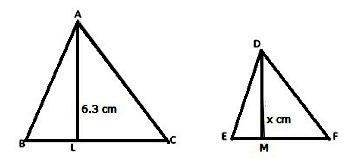We know that the ratio of the area of two similar triangles is equal to the ratio of the square of corresponding altitudes:

$\frac{ar(\Delta ABC)}{ar(\Delta DEF)}=\frac{AL^{2}}{DM^{2}}$

$\Rightarrow$ $\frac{81}{49}=\frac{(6.3)^{2}}{x^{2}}$

$\Rightarrow$ x2 = $\left ( \frac{49\times 6.3\times 6.3}{81} \right )=24.01$

$\Rightarrow$ x = $\sqrt{24.01}=4.9cm$

Hence, DM = 4.9 cm

Therefore, the required altitude is 4.9 cm

Question 2:The areas of two similar triangles are 100 cm2 and 64 cm2 respectively. If a median of the smaller triangle is 5.6 cm, find the corresponding median of the other.

Solution:

Given: $\Delta$ACB ~ $\Delta$DEF such that ar($\Delta$ABC) = 100 cm2 and ar($\Delta$DEF) = 64 cm2

Let AP and DQ be the corresponding medians of $\Delta$ABC and $\Delta$DEF respectively such that DQ = 5.6 cm.

Let AP = x cm.

We know that the ratio of the areas of two similar triangles is equal to the ratio of the squares of their corresponding medians.

Therefore, $\frac{area of \Delta ABC}{area of \Delta DEF}=\frac{AP^{2}}{DQ^{2}}$

$\Rightarrow$ $\frac{100}{64}=\frac{ x^{2}}{ (5.6)^{2}}$

$\Rightarrow$ x2 = $\frac{100\times (5.6)^{2}}{64}$

$\Rightarrow$ x = $\sqrt{\frac{100\times (5.6)^{2}}{64}}$

$\Rightarrow$ x = $\frac{10\times 5.6}{8}=7cm$

Hence, AP = 7 cm

Question 3:In the given figure, ABC is a triangle and PQ is a straight line meeting AB in P and AC in Q. If AP = 1 cm, PB = 3 cm, AQ = 1.5 cm, QC = 4.5 cm, prove that area of $\Delta$APQ is $\frac{1}{16}$ of the area of $\Delta$ABC.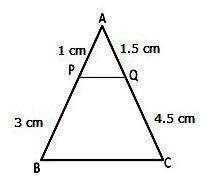Solution:

Given: AP = 1 cm, PB = 3 cm, AQ = 1.5 cm, QC = 4.5 cm

AB = AP + PB = (1 + 3) cm = 4 cm

AC = AQ + QC = (1.5 + 4.5) cm = 6 cm

Now, we have:

$\frac{AP}{AB}=\frac{1}{4}$, $\frac{AQ}{QC}=\frac{1.5}{6}=\frac{1}{4}$

In $\Delta$APQ and $\Delta$ABC, we have

$\angle$APQ = $\angle$ABC (corresponding angles)

$\angle$AQP = $\angle$ACB (corresponding angles)

$\Delta$APQ ~ $\Delta$ABC [by AA similarity]

$\Rightarrow$ $\frac{ar(\Delta APQ)}{ar(\Delta ABC)}=\frac{AP^{2}}{AB^{2}}$

$\Rightarrow$ $\frac{ar(\Delta APQ)}{ar(\Delta ABC)}=\left ( \frac{AP}{AB} \right )^{2}$

$\Rightarrow$ $\frac{ar(\Delta APQ)}{ar(\Delta ABC)}=\left ( \frac{1}{4} \right )^{2}=\frac{1}{16}$

$\Rightarrow$ $ar(\Delta APQ)=\frac{1}{16}ar(\Delta ABC)$

Hence proved.

Question 4:In the given figure, DE || BC. If DE = 3 cm, BC = 6 cm and ar($\Delta$ADE) = 15 cm2, find the area of $\Delta$ABC.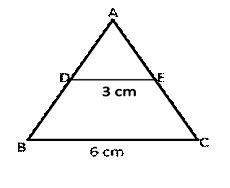Solution:

Given DE || BC

DE = 3 cm and BC = 6 cm

ar($\Delta$ADE) = 15 cm2

In $\Delta$ADE and $\Delta$ABC, we have

$\angle$ADE = $\angle$ABC (corresponding angles)

$\angle$AED = $\angle$ACB (corresponding angles)

Therefore, $\Delta$ADE ~ $\Delta$ABC (by AA similarity)

$\Rightarrow$ $\frac{ar(\Delta ADE)}{ar(\Delta ABC)}=\frac{DE^{2}}{BC^{2}}$

$\Rightarrow$ $\frac{15}{ar(\Delta ABC)}=\frac{3^{2}}{6^{2}}=\frac{9}{36}$

$\Rightarrow$ $ar(\Delta ABC)=\frac{15\times 36}{9}=60cm^{2}$

Therefore, $ar(\Delta ABC)=60cm^{2}$

Question 5:$\Delta$ABC is right-angled at A and AD $\perp$ BC. If BC = 13 cm and AC = 5 cm, find the ratio of the areas of $\Delta$ABC and $\Delta$ADC.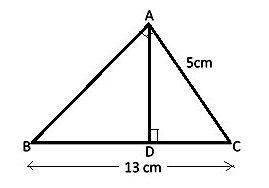Solution:

In $\Delta$BAC and $\Delta$ADC = 90o (AD $\perp$ BC)

$\angle$ACB = $\angle$DCA (common)

$\Delta$ BAC ~ $\Delta$ADC

$\frac{ar(\Delta ABC)}{ar(\Delta ADC)}=\frac{ar(\Delta BAC)}{ar(\Delta ADC)}=\frac{BC^{2}}{AC^{2}}$

$\Rightarrow$ $\frac{ar(\Delta ABC)}{ar(\Delta ADC)}=\frac{13^{2}}{5^{2}}=\frac{169}{25}$

Therefore, the ratio of the areas of $\Delta$ABC and $\Delta$ADC = 169 : 25

Question 6:In the given figure, DE || BC and DE : BC = 3 : 5. Calculate the ratio of the areas of $\Delta$ADE and the trapezium BCED.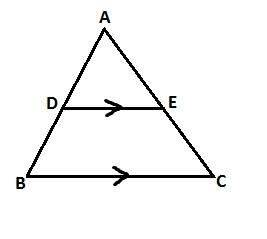Solution:

Let DE = 3x and BC = 5x

In $\Delta$ADE and $\Delta$ABC, we have

$\angle$ADE = $\angle$ABC (corresponding angles)

$\angle$AED = $\angle$ACB (corresponding angles)

$\Delta$ADE ~ $\Delta$ABC (by AA similarity)

$\Rightarrow$ $\frac{ar(\Delta ADE)}{ar(\Delta ABC)}=\frac{DE^{2}}{BC^{2}}=\left ( \frac{3x}{5x} \right )^{2}=\frac{9x^{2}}{25x^{2}}$

Let, ar($\Delta$ADE) = 9x2 units

Then, ar($\Delta$ABC) = 25x2 units

Therefore, $ar(trap.BCED)=ar(\Delta ABC)-ar(\Delta ADE)=(25x^{2}-9x^{2})=16x^{2}units$

Therefore, $\frac{ar(\Delta ADE)}{ar(trap.BCED)}=\frac{9x^{2}}{16x^{2}}=\frac{9}{16}$

Therefore, ratio of ar($\Delta$ADE) to the ar(trap BCED) = 9 : 16

Question 7:In $\Delta$ABC, D and E are the midpoints of AB and AC respectively. Find the ratios of the areas of $\Delta$ADE and $\Delta$ABC.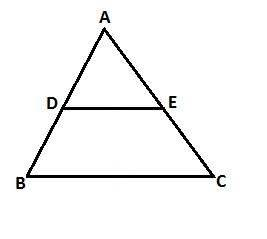Solution:

In $\Delta$ABC, D, and E are midpoint of AB and AC respectively.

So, DE || BC and DE = ½ BC

Now, in $\Delta$ADE and $\Delta$ABC, we have

$\angle$ADE = $\angle$ABC (corresponding angles)

$\angle$AED = $\angle$ACB (corresponding angles)

$\Delta$ADE ~ $\Delta$ABC (by AA similarity)

Let AD = x and AB = 2x

Therefore, $\frac{ar(\Delta ADE)}{ar(\Delta ABC)}=\frac{AD^{2}}{AB^{2}}$

$\Rightarrow$ $\frac{ar(\Delta ADE)}{ar(\Delta ABC)}=\frac{x^{2}}{(2x)^{2}}=\frac{1}{4}$

Therefore, the ratio of the areas of $\Delta$ADE and $\Delta$ABC = 1 : 4

## EXERCISE 4D

Question 1:The sides of certain triangles are given below. Determine which of them are right angles.

(i) 9 cm, 16 cm, 18 cm

(ii) 7 cm, 24 cm, 25 cm

(iii) 1.4 cm, 4.8 cm, 5 cm

(iv) 1.6 cm, 3.8 cm, 4 cm

(v)(a – 1) cm, $2\sqrt{a}$ cm, (a + 1) cm

Solution:

For a given triangle to be a right angled, the sum of the squares of the two sides must be equal to the square of the largest side.

(i)Let a = 9 cm, b = 16 cm and c = 18 cm. Then

(a2 + b2) = [92 + (16)2] = (81 + 256) cm2 = 337 cm2

And c2 = (18)2 cm2 = 324 cm2

Therefore, (a2 + b2) $\neq$ c2

Hence, the given triangle is not right angled.

(ii)Let a = 7 cm, b = 24 cm and c = 25 cm, Then

(a2 + b2) = [72 + (24)2] = (49 + 576) cm2 = 625 cm2

And c2 = (25)2 cm2 = 625 cm2

Therefore, (a2 + b2) = c2

Hence, the given triangle is a right triangle.

(iii)Let a = 1.4 cm, b = 4.8 cm, and c = 5 cm

(a2 + b2) = [(1.4)2 + (4.8)2] = (1.96 + 23.04) cm2 = 25 cm2

And c2 = (5)2 cm2 = 25 cm2

Therefore, (a2 + b2) = c2

Hence, the given triangle is a right triangle.

(iv)Let a = 1.6 cm, b = 3.8 cm and c = 4 cm

(a2 + b2) = [(1.6)2 + (3.8)2] = (2.56 + 14.44) cm2 = 17 cm2

And c2 = (4)2 cm2 = 16 cm2

Therefore, (a2 + b2) $\neq$ c2

Hence, the given triangle is not a right triangle.

(v)Let p = (a – 1) cm, q = $2\sqrt{a}$ cm and r = (a + 1) cm

(p2 + q2) = [(a – 1)2 + ($2\sqrt{a}$)2] = (a2 + 1 – 2a + 4a) cm2 = (a + 1)2 cm2

And r2 = (a + 1)2 cm2

Therefore, (p2 + q2) = r2

Hence, the given triangle is a right triangle.

Question 2:A man goes 80 m due east and then 150 m due north. How far is he from the starting point?

Solution:

Starting from A, let the man goes from A to B and from B to C, as shown in the figure.

Then,

AB = 80 m, BC = 150 m and $\angle$ABC = 90o

From right $\Delta$ABC, we have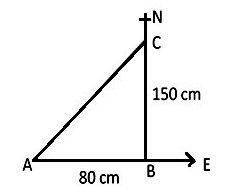By Pythagoras theorm, we have:

AC2 = (AB2 + BC2) m2

= [(80)2 + (150)2] m2 = (6400 + 22500) m2 = 28900 m2

Therefore, AC = $\sqrt{28900}$ m = 170 m

Hence, the man is 170 m north-east from the starting point.

Question 3:A man goes 10 m due south and then 24 m due west. How far is he from the starting point?

Solution:

Starting from O, let the man goes from O to A and then A to B as shown in the figure.

Then,

OA = 10 m, AB = 24 m and $\angle$OAB = 90o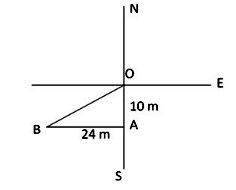Using Pythagoras theorem:

OB2 = OA2 + AB2

$\Rightarrow$ OB2 = 102 + 242

$\Rightarrow$ OB2 = 100 + 576

$\Rightarrow$ OB2 = 676

$\Rightarrow$ OB = $\sqrt{676}$ = 26 m

Hence, the man is 26 m south-west from the starting position.

Question 4:A 13-m-long ladder reaches a window of a building 12 m above the ground. Determine the distance of the foot of the ladder from the building.

Solution:

Let AB be the building and CB be the ladder.

Then,

AB = 12 m, CB = 13 m and $\angle$CAB = 90o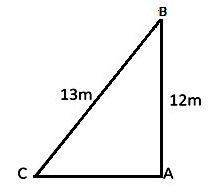By Pythagoras theorem, we have:

CB2 = AB2 + AC2

AC2 = [CB2 – AB2] = [(13)2 – (12)2] m2 = (169 – 144) m2 = 25 m2

$\Rightarrow$ AC = $\sqrt{25}$ m = 5 m

Hence, the distance of the foot of the ladder from the building is 5 m.

Question 5:A ladder is placed in such a way that its foot is at a distance of 15 m from a wall and its top reaches a window 20 m above the ground. Find the length of the ladder.

Solution:

Let AB be the wall where window is at B, CB be the ladder and AC be the distance between the foot of the ladder and wall.

Then,

AB = 20 m, AC = 15 m, and $\angle$CAB = 90o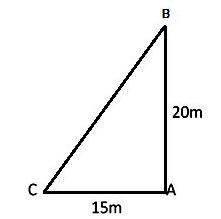By Pythagoras theorem, we have

CB2 = AB2 + AC2 = [(20)2 + (15)2] m2 = (400 + 225) m2 = 625 m2

CB = $\sqrt{625}$ m = 25 m

Hence, the length of ladder is 25 m.

Question 6: Two vertical poles of height 9 m and 14 m stand on a plane ground. If the distance between their feet is 12 m, find the distance between their tops.

Solution:

Let AB and CD be the given vertical poles.

Then,

AB = 9 m, CD = 14 m and AC = 12 m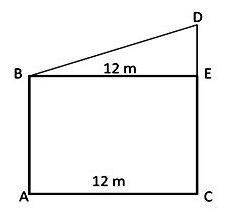Const:Draw, BE || AC.

Then,

CE = AB = 9 m and BE = AC = 12 m

DE = (CD – CE) = (14 – 9) = 5 m

In right $\Delta$BED, we have

BD2 = BE2 + DE2 = [(12)2 + (5)2] m2 = (144 + 25) m2 = 169 m2

$\Rightarrow$ BD = $\sqrt{169}$ = 13 m

Hence, the distance between their tops is 13 m.

Question 7:In the given figure, O is a point inside a $\Delta$PQR such that $\angle$QPR = 90o, OP = 6 cm and OR = 8 cm. If PQ = 24 cm and QR = 26 cm, prove that $\Delta$PQR is right-angled.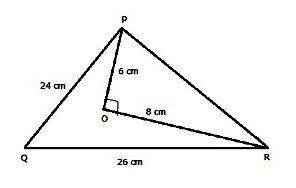Solution:

In $\Delta$PQR, $\angle$QPR = 90o, PQ = 24 cm, and QR = 26 cm2

In $\Delta$POR, PO = 6 cm, QR = 8 cm and $\angle$POR = 90o

In $\Delta$POR,

PR2 = PO2 + OR2

PR2 = (62 + 82) cm2 = (36 + 64) cm2 = 100 cm2

PR = $\sqrt{100}$ cm = 10 cm

In $\Delta$PQR,

By Pythagoras theorem, we have

QR2 = QP2 + PR2

(26)2 cm2 = (242 + 102) cm2

676 cm2 = (576 + 100) cm2

676 cm2 = 676 cm2

Hence, QR2 = QP2 + PR2

(Sum of square of two sides equal to square of the greatest side)

Hence, $\Delta$PQR is a right triangle which is right angled at P.

Question 8:$\Delta$ABC is an equilateral triangle of side 2a units. Find each of its altitudes.

Solution:

In an equilateral triangle all sides are equal.

Then, AB = BC = AC = 2a units

Const: Draw an altitude AD $\perp$ BC

Given BC = 2a. Then, BD = a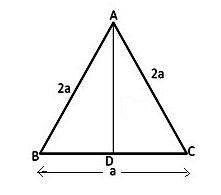In $\Delta$ABD,

$\angle$ADB = 90o

(AB)2 = (AD)2 + (BD)2 [by Pythagoras thorem]

= [(2a)2 – (a)2] sq. unit = (4a2 – a2) sq. unit

AD = $\sqrt{3a^{2}}$ unit = $a\sqrt{3}$ unit.

Question 9:Find the height of an equilateral triangle of side 12 cm.

Solution:

$\Delta$ABC is an equilateral triangle in which all side are equal.

Therefore, AB = BC = AC = 12 cm

If BC = 12 cm

Then, BD = DC = 6 cm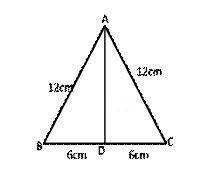In $\Delta$ADB,

AD2 = [(12)2 – (6]2] cm2

AD = $\sqrt{108}$ cm = $6\sqrt{3}$ cm

Hence, the height of the triangle is $6\sqrt{3}$ cm.

Question 10:Find the length of a diagonal of a rectangle whose adjacent sides are 30 cm and 16 cm.

Solution:

Let ABCD is the given rectangle, let BD is a diagonal making a $\Delta$ADB.

$\Rightarrow$ $\angle$BAD = 90o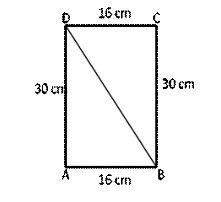Using Pythagoras theorem:

DB2 = (162 + 302) cm2

DB = $\sqrt{16^{2}+30^{2}}$ cm = $\sqrt{256+900}$ = 34 cm

Hence, length of diagonal DB is 34 cm.

Question 11:Find the length of each side of a rhombus whose diagonals are 24 cm and 10 cm long.

Solution:

Let ABCD be the given rhombus whose diagonals intersect at O.

Then AC = 24 cm and BD = 10 cm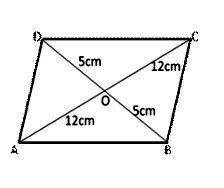We know that the diagonals of a rhombus bisect each other at right angles.

OA = ½ AC = 12 cm

OB = ½ BD = 5 cm

And $\angle$AOB = 90o

From right $\Delta$AOB, we have

AB2 = OA2 + OB2

$\Rightarrow$ AB2 = [(12)2 + (5)2] cm2 = (144 + 25) cm2 = 169 cm2

$\Rightarrow$ AB = $\sqrt{169}$ cm = 13 cm

Hence, each side of a rhombus is 13 cm.

Question 12:In $\Delta$ABC, D is the midpoint of BC and AE $\perp$ BC. If AC > AB, show that

AB2 = AD2 – BC.DE + $\frac{1}{4}$BC2.

Solution:

Given:$\Delta$ABC in which D is the midpoint of BC. AE $\perp$ BC and AC > AB.

Then, BD = CD and $\angle$AED = 90o

Then, $\angle$ADE < 90o and $\angle$ADC > 90o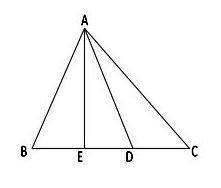In $\Delta$AED,

$\angle$AED = 90o

Therefore, AD2 = AE2 + DE2

$\Rightarrow$ AE2 = (AD2 – DE2) ——- (1)

In $\Delta$AEB,

$\angle$AEB = 90o

Therefore, AB2 = AE2 + BE2 ——- (2)

Putting the value of AE2 from (1) in (2), we get

Therefore, AB2 = (AD2 – DE2) + BE2

= (AD2 – DE2) + (BD2 – DE2) [But BD = ½ BC]

= AD2 – DE2 + $\left ( \frac{1}{2}BC-DE \right )^{2}$

= $AD^{2}-DE^{2}+\frac{1}{4}BC^{2}+DE^{2}-BC.DE$

AB2 = AD2 – BC.DE + $\frac{1}{4}$BC2

Question 13: In the given figure, D is the midpoint of side BC and AE $\perp$ BC. If BC = a, AC = b, AB = c, ED = x, AD = p and AE = h, prove that

(i) b2 = p2 + ax + $\frac{a^{2}}{4}$

(ii) c2 = p2 – ax + $\frac{a^{2}}{4}$

(iii) (b2 + c2) = 2p2 + $\frac{1}{2}$a2

(iv) (b2 – c2) = 2ax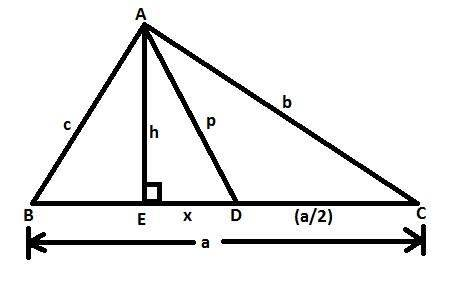Solution:

Given: D is the midpoint of side BC, AE $\perp$ BC, BC = a, AC = b, AB = c, ED = x, AD = p and AE = h

In $\Delta$AEC, $\angle$AEC = 90o

AD2 = 2AE2 + ED2 (by Pythagoras theorem)

$\Rightarrow$ p2 = h2 + x2

(i)In $\Delta$AEC, $\angle$AEC = 90o

$b^{2}=h^{2}+\left ( x+\frac{a}{2} \right )^{2}=(h^{2}+x^{2})+ax+\frac{a^{2}}{4}$

Therefore, b2 = p2 + ax + $\frac{a^{2}}{4}$ ——- (1)

(ii)In $\Delta$ABE, $\angle$ABE = 90o

AB2 = AE2 + BE2 (by pythagoras theorem)

$\Rightarrow$ $c^{2}=h^{2}+\left ( \frac{a}{2}-x \right )^{2}$ ——— (2)

= $(h^{2}+x^{2})-ax+\frac{a^{2}}{4}$

= $p^{2}-ax+\frac{a^{2}}{4}$

Hencxe, c2 = p2 – ax + $\frac{a^{2}}{4}$

(iii)Adding (1) and (2), we get:

$b^{2}+c^{2}=p^{2}+ax+\frac{a^{2}}{4}+p^{2}-ax+\frac{a^{2}}{4}$

$(b^{2}+c^{2})=2p^{2}+\frac{1}{2}a^{2}$

(iv)Subtracting (2) from (1), we get:

$b^{2}-c^{2}=p^{2}+ax+\frac{a^{2}}{4}-\left ( p^{2}-ax+\frac{a^{2}}{4} \right )$

= $p^{2}+ax+\frac{a^{2}}{4}-p^{2}+ax-\frac{a^{2}}{4}$

$(b^{2}-c^{2})=2ax$

Question 14:In $\Delta$ABC, AB = AC. Side BC is produced to D. Prove that

Solution: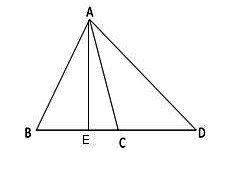Const:Draw a perpendicular AE from A

Thus, AE $\perp$ BC

Proof:

In $\Delta$ABC, AB = AC

And AE is a bisector of BC

Then, BE = EC

In right angle triangles $\Delta$AED and $\Delta$ACE

AD2 = AE2 + DE2 —— (1)

AC2 = AE2 + CE2 ——- (2)

Subtracting (2) from (1),

$\Rightarrow$ (AD2 – AC2) = DE2 – CE2

$\Rightarrow$ AD2 – AC2 = (DE + CE) (DE – CE) = (DE + BE) (DE – CE) [CE = BE]

$\Rightarrow$ AD2 – AC2 = BD x CD

Hence proved

### Key Features of RS Aggarwal Class 10 Solutions Chapter 4 – Triangles Ex 4B (4.2)

• The solutions has been made by experienced subject experts based on syllabus and guidelines issued by CBSE and NCERT.
• It improves your performance level, speed and accuracy.
• The RS Aggarwal Solutions for Class 10 can be referred by you while solving the exercise questions.